#The Wolfram Language:Fast Introduction for Programmers

All sections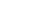Get Started

# PatternsVideo Version

Notes for Java programmers:

The Wolfram Language's pattern language lets you describe patterns for arbitrary symbolic structures, allowing powerful regex-like manipulation to be generalized to any expression and any form of data.

Notes for Python programmers:

The Wolfram Language's pattern language lets you describe patterns for arbitrary symbolic structures, allowing powerful regex-like manipulation to be generalized to any expression and any form of data.

Patterns stand for classes of expressions. The basic pattern construct _ (pronounced "blank") stands for any expression.

Find cases in a list matching the pattern f[_]:

In:=1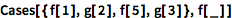`Cases[{f, g, f, g}, f[_]]`
Out=1x_ (short for x:_) stands for a pattern whose value will be named x: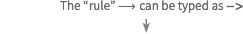In:=2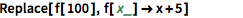`Replace[f, f[x_] -> x + 5]`
Out=2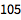/. means "replace everywhere":

In:=3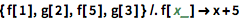`{f, g, f, g} /. f[x_] -> x + 5`
Out=3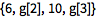Notes for Java programmers:

The sort of structural metaprogramming shown here is unique to the Wolfram Language's symbolic structure.

Notes for Python programmers:

The structural metaprogramming shown here, which treats code and data in the same way, is unique to the Wolfram Language's symbolic structure.

__ ("double blank") stands for any sequence of expressions:

In:=1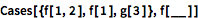`Cases[{f[1, 2], f, g}, f[__]]`
Out=1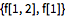a | b | c stands for a, b or c:

In:=1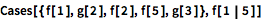`Cases[{f, g, f, f, g}, f[1 | 5]]`
Out=1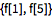Notes for Java programmers:

This usage of | resembles Java's regex notation, although in Java | can also indicate a bitwise OR.

Notes for Python programmers:

| is used in Wolfram Language patterns similarly to its use in Python's regular expressions. However, in the Wolfram Language, | works for any type of symbolic pattern matching, not just for strings.

In:=2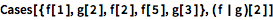`Cases[{f, g, f, f, g}, (f | g)]`
Out=2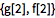_h stands for any expression with head h:

In:=1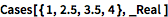`Cases[{1, 2.5, 3.5, 4}, _Real]`
Out=1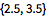:> is a delayed rule—the analog of := for a rule.

QUICK REFERENCE: Operations Involving Patterns

Which of these patterns matches the expression g[1, 2, 3]?

Which of these picks out all elements from the list {f, g, f, g} matching g[_]?

The result of the expression {f, f, f[x]} /. f[x_] `→` x + 4 is: